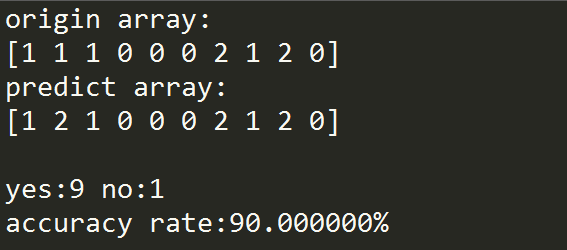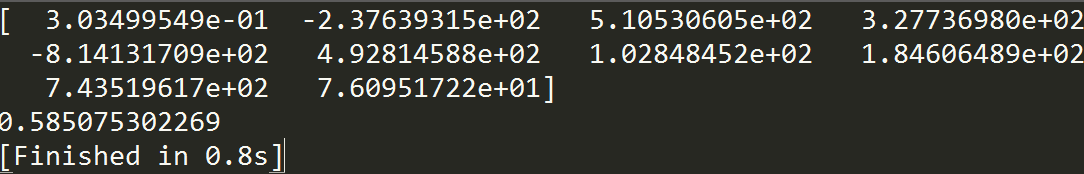# sklearn学习（3）针对科学数据处理的统计学习教程{未完}

http://scikit-learn.org/stable/tutorial/statistical_inference/index.html

http://www.cnblogs.com/taceywong/p/4570155.html  个人根据实际情况有删改

## 一、统计学习：scikit-learn中的设置与评估函数对象

### （1）数据集

scikit-learn 从二维数组描述的数据中学习信息。他们可以被理解成多维观测数据的列表。如（n,m），n表示样例轴，y表示特征轴。

```>>>from sklearn import datasets
>>>data = iris.data
>>>data.shape
(150, 4)```

digits数据集由1797个8×8手写数字图片组成

```>>>digits = datasets.load_digits()
>>>digits.images.shape
(1797, 8, 8)
>>>import pylab as pl
>>>pl.imshow(digits.images[-1], cmap=pl.cm.gray_r)
<matplotlib.image.AxesImage object at ...>
``````python
>>>data = digits.images.reshape((digits.images.shape,-1))```

### (2)估计函数对象

`>>>estimator.fit(data)`

```>>>estimator = Estimator(param1=1, param2=2)
>>>estimator.param1
1```

`>>>estimator.estimated_param_`

## 二、有监督学习：从高维观察数据预测输出变量

### （1）近邻和高维灾难

iris分类
iris分类是根据花瓣、萼片长度、萼片宽度来识别三种不同类型的iris的分类任务:

```>> import numpy as np
>> from sklearn import datasets
>> iris_X = iris.data
>> iris_y = iris.target
>> np.unique(iris_y)
array([0, 1, 2])```

#coding=utf-8   含有中文必须规定编码格式
import numpy as np
from sklearn import datasets
def count(a1,a2,yes,no):#统计正确数与错误数
i=0
while i<a1.size:
if a1[i]!=a2[i]:
no+=1
else :
yes+=1
i+=1
return yes,no
def calRate(origin,predict):
print "origin array:\n%s"%(origin)
print "predict array:\n%s"%(predict)
yes,no=0,0
yes,no=count(origin,predict,yes,no)
rate=float(100*yes)/float(yes+no)#正确率
print "\nyes:%d no:%d \naccuracy rate:%f%%\n"%(yes,no,rate)

if __name__ == "__main__":
iris_X=iris.data
iris_Y=iris.target

np.random.seed(0)
indices=np.random.permutation(len(iris_X))
iris_X_train=iris_X[indices[:-10]]
iris_Y_train=iris_Y[indices[:-10]]
iris_X_test=iris_X[indices[-10:]]
iris_Y_test=iris_Y[indices[-10:]]

from sklearn.neighbors import KNeighborsClassifier
knn=KNeighborsClassifier()
knn.fit(iris_X_train,iris_Y_train)
predict=knn.predict(iris_X_test)
origin=iris_Y_test
calRate(origin,predict)### （2）线性模型：从回归到稀疏性

```Diabets数据集（糖尿病数据集）

diabetes_X_train = diabetes.data[:-20]
diabetes_X_test = diabetes.data[-20:]
diabetes_y_train = diabetes.target[:-20]
diabetes_y_test = diabetes.target[-20:]

【线性回归】的最简单形式给数据集拟合一个线性模型，主要是通过调整一系列的参数以使得模型的残差平方和尽量小

```线性模型：y = βX+b
X:数据
y：目标变量
β：回归系数
b:观测噪声（bias，偏差）```from sklearn import linear_model
regr = linear_model.LinearRegression()
regr.fit(diabetes_X_train, diabetes_y_train)
print(regr.coef_)
np.mean((regr.predict(diabetes_X_test)-diabetes_y_test)**2)#均方误差
print(regr.score(diabetes_X_test, diabetes_y_test))#1代表最佳预测，0表示x与y没有线性关系## 发布者